# TypeError: argument of type 'int' is not iterable (Python)11 min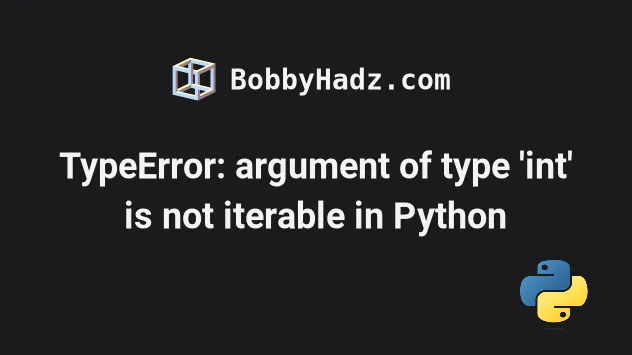Photo from Unsplash

Make sure to click on the correct subheading depending on your error message.

## TypeError: argument of type 'int' is not iterable in Python#

The Python "TypeError: argument of type 'int' is not iterable" occurs when we use the membership test operators (in and not in) with an integer value.

To solve the error, correct the assignment or convert the int to a string.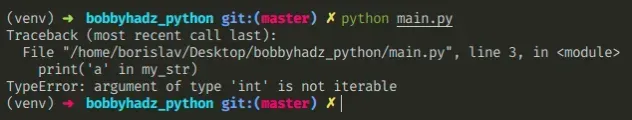Here is an example of how the error occurs.

main.py
```Copied!```my_str = 15

# ⛔️ TypeError: argument of type 'int' is not iterable
print('a' in my_str)
``````

We tried to use a membership test operator with an integer value and got the error.

Chances are you meant to use the operator with an iterable, e.g. a string or a list.

## Convert the integer to a string or use a list#

One way to solve the error is to convert the integer to a string.

main.py
```Copied!```my_str = 15

print('a' in str(my_str))  # 👉️ False

print('5' in str(my_str))  # 👉️ True
``````

Strings are iterable, so converting the integer to a string solves the issue.

## Track down where the variable got assigned an integer#

However, the best way to solve the error is to track down where the variable got assigned an int and correct the assignment.

For example, you can reassign the variable if it stores a value of an unexpected type.

main.py
```Copied!```my_list = 15

if not isinstance(my_list, list):
my_list = []

print('a' in my_list)  # 👉️ False
``````

We check if the `my_list` variable doesn't store a list, and if it doesn't, we set it to an empty list before using the `in` operator.

You can use this approach with any other object, e.g. `str`, `dict`, `tuple`, etc.

main.py
```Copied!```my_str = 15

if not isinstance(my_str, str):
my_str = ""

print('a' in my_str)  # 👉️ False
``````

If the variable is not a string, we set it to an empty string.

## Check if the value is not an integer before using `in`#

Alternatively, you can check if the value is not an `int` before using the `in` or `not in` operators.

main.py
```Copied!```my_str = 15

if not isinstance(my_str, int):
print('a' in my_str)
else:
# 👇️ this runs
print('value is an integer')
``````

We check if the value is not an instance of the `int` class, and if it isn't, we use the `in` operator to test for membership.

However, it's safer to check if the value is of the expected type, e.g. a `str`, a `list`, or a `dict`.

main.py
```Copied!```if isinstance(my_list, list):
print('a' in my_list)
else:
print('value is not a list')
``````

Our `if` statement checks if the value is an instance of the `list` class, and if it is, we use the `in` operator.

The in operator tests for membership. For example, `x in s` evaluates to `True` if `x` is a member of `s`, otherwise it evaluates to `False`.

main.py
```Copied!```my_str = 'hello world'

print('world' in my_str)  # 👉️ True

print('another' in my_str)  # 👉️ False
``````

`x` not in `s` returns the negation of `x` in `s`.

All built-in sequences and set types support the `in` and `not in` operators.

When used with a dictionary, the operators check for the existence of the specified key in the `dict` object.

The Python "TypeError: argument of type 'int' is not iterable" occurs when we use the membership test operators (in and not in) with an integer value.

To solve the error, correct the assignment or convert the int to a string.

## TypeError: 'numpy.int64' object is not iterable in Python#

The Python "TypeError: 'numpy.int64' object is not iterable" occurs when we try to iterate over an integer or pass an integer to a built-in function like `sum()` or `min()`.

To solve the error, iterate over an array of integers or pass an iterable to built-in methods.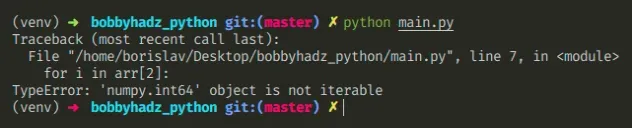Here is an example of how the error occurs.

main.py
```Copied!```import numpy as np

my_int = np.int64(25)

# ⛔️ TypeError: 'numpy.int64' object is not iterable
for i in my_int:
print(i)
``````

We tried to iterate over a numpy integer value which caused the error.

Hre is another example of how the error occurs.

main.py
```Copied!```import numpy as np

arr = np.array([1, 2, 3])

# ⛔️ TypeError: 'numpy.int64' object is not iterable
for i in arr:
print(i)
``````

The array element at index `2` is an integer, so we can't iterate over it.

## Iterating over a range of numpy integers#

We can use the `range()` built-in function to iterate over a range.

main.py
```Copied!```import numpy as np

arr = np.array([1, 2, 3])

for i in range(arr):
print(i)  # 👉️ 0, 1, 2
``````

If you need to iterate over an array, use a basic for loop.

main.py
```Copied!```import numpy as np

arr = np.array([1, 2, 3])

for i in arr:
print(i)  # 👉️ 1, 2, 3
``````

The range function is commonly used for looping a specific number of times in `for` loops and takes the following parameters:

NameDescription
`start`An integer representing the start of the range (defaults to `0`)
`stop`Go up to, but not including the provided integer
`step`Range will consist of every N numbers from `start` to `stop` (defaults to `1`)

## Passing an integer to functions that expect an iterable#

Another common cause of the error is passing an integer to the built-in constructors, e.g. `list()`, `dict()`, `tuple()` and `set()`.

Here is an example.

main.py
```Copied!```import numpy as np

int_1 = np.int64(25)

int_2 = np.int64(50)

# ⛔️ TypeError: 'numpy.int64' object is not iterable
result = sum(int_1, int_2)
``````

Functions like `sum()`, `max()` and `min()` take an iterable as an argument, so we can't pass it numpy integers directly.

Instead, wrap the numpy integers into a list or an array.

main.py
```Copied!```import numpy as np

int_1 = np.int64(25)

int_2 = np.int64(50)

result = sum([int_1, int_2])

print(result) # 👉️ 75
``````

Alternatively, you can use a numpy array.

main.py
```Copied!```import numpy as np

int_1 = np.int64(25)

int_2 = np.int64(50)

arr = np.array([int_1, int_2])

result = sum(arr)

print(result)  # 👉️ 75
``````

The following 4 calls to the built-in constructors cause the error.

main.py
```Copied!```import numpy as np

arr = np.array([1, 2, 3])

# ⛔️ TypeError: 'numpy.int64' object is not iterable
list(arr)

dict(arr)

tuple(arr)

set(arr)
``````

To solve the error, we have to correct the assignment and figure out where the integer value is coming from.

Here are working examples of using the 4 built-ins.

main.py
```Copied!```l = list(['a', 'b', 'c'])
print(l)  # 👉️ ['a', 'b', 'c']

print(d)  # 👉️ {'name': 'Bobby Hadz', 'age': 30}

t = tuple([1, 2, 3])
print(t)  # 👉️ (1, 2, 3)

s = set(['a', 'b', 'a'])
print(s)  # 👉️ {'a', 'b'}
``````

You have to figure out where the integer value came from and correct the assignment.

If you need to iterate with both the index and the current item, use the `enumerate()` function.

main.py
```Copied!```import numpy as np

arr = np.array([1, 2, 3])

for idx, el in enumerate(arr):
print(idx, el)  # 👉️ 0 1, 1 2, 2 3
``````

If you need to check if an object is iterable, use a `try/except` statement.

main.py
```Copied!```my_str = 'hello'

try:
my_iterator = iter(my_str)

for i in my_iterator:
print(i) # 👉️ h, e, l, l, o
except TypeError as te:
print(te)
``````

The iter() function raises a `TypeError` if the passed-in value doesn't support the `__iter__()` method or the sequence protocol (the `__getitem__()` method).

If we pass a non-iterable object like a numpy integer to the `iter()` function, the `except` block is run.

main.py
```Copied!```import numpy as np

arr = np.array([1, 2, 3])

try:
my_iterator = iter(arr)

for i in my_iterator:
print(i)
except TypeError as te:
print(te)  # 👉️ 'numpy.int64' object is not iterable
``````

Examples of iterables include all sequence types (`list`, `str`, `tuple`) and some non-sequence types like `dict`, file objects and other objects that define an `__iter__()` or a `__getitem__()` method.

The Python "TypeError: 'numpy.int64' object is not iterable" occurs when we try to iterate over an integer or pass an integer to a built-in function like `sum()` or `min()`.

To solve the error, iterate over an array of integers or pass an iterable to built-in methods.

## TypeError: 'int' object is not iterable in Python#

The Python "TypeError: 'int' object is not iterable" occurs when we try to iterate over an integer or pass an integer to a built-in function like, `sum()`, `list()` or `tuple()`.

To solve the error, use the `range()` built-in function to iterate over a range or pass a list of integers to the function.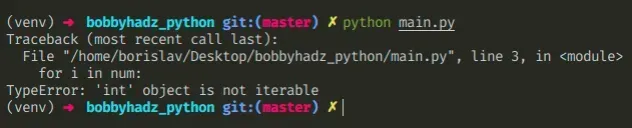Here is an example of how the error occurs.

main.py
```Copied!```num = 10

# ⛔️ TypeError: 'int' object is not iterable
for i in num:
print(i)
``````

We are trying to iterate over an integer, but integers are not iterable.

## Iterating over a range#

We can use the `range()` built-in function to iterate over a range.

main.py
```Copied!```num = 10

for i in range(num):
print(i) # 👉️ 0, 1, 2, ... 8, 9
``````

The range function is commonly used for looping a specific number of times in `for` loops and takes the following parameters:

NameDescription
`start`An integer representing the start of the range (defaults to `0`)
`stop`Go up to, but not including the provided integer
`step`Range will consist of every N numbers from `start` to `stop` (defaults to `1`)

If you only pass a single argument to the `range()` constructor, it is considered to be the value for the `stop` parameter.

If values for the `start` and `stop` parameters are provided, the `start` value is inclusive, whereas the `stop` value is exclusive.

main.py
```Copied!```num = 5

for i in range(1, num):
print(i)  # 👉️ 1, 2, 3, 4
``````

## Handling the error in a `try/except`#

If you need to handle the error, use a `try/except` statement.

main.py
```Copied!```num = 5

try:
for i in num:
print(i)
except TypeError:
# The object is not iterable
# <class 'int'>
print('The object is not iterable')
print(type(num))
``````

The `try` statement tries to iterate over the value and if an exception is raised, the `except` block runs.

You can print the value and its type in the `except` block to debug your code.

## Calling a built-in function with an integer instead of an iterable#

The error is also caused if we pass an integer to built-in functions like `sum()`, `max()` and `min()`.

main.py
```Copied!```int_1 = 10
int_2 = 20

# ⛔️ TypeError: 'int' object is not iterable
result = sum(int_1, int_2)
``````

These functions take an iterable as an argument and cannot be called with an integer directly.

Instead, pass a list containing the integers as an argument to the function.

main.py
```Copied!```int_1 = 10
int_2 = 20

# ⛔️ TypeError: 'int' object is not iterable
result = sum([int_1, int_2])
print(result) # 👉️ 30
``````

Another common cause of the error is passing an integer to the built-in constructors, e.g. `list()`, `dict()`, `tuple()` and `set()`.

The following 4 calls to the built-in constructors cause the error.

main.py
```Copied!```num = 10

# ⛔️ TypeError: 'int' object is not iterable
list(num)

dict(num)

tuple(num)

set(num)
``````

To solve the error, we have to correct the assignment and figure out where the integer value is coming from.

Here are working examples of using the 4 built-ins.

main.py
```Copied!```l = list(['a', 'b', 'c'])
print(l)  # 👉️ ['a', 'b', 'c']

print(d)  # 👉️ {'name': 'Bobby Hadz', 'age': 30}

t = tuple([1, 2, 3])
print(t)  # 👉️ (1, 2, 3)

s = set(['a', 'b', 'a'])
print(s)  # 👉️ {'a', 'b'}
``````

You have to figure out where the integer value came from and correct the assignment.

## The error is commonly caused when using `len()`#

The error is commonly caused when using the `len()` built-in function.

main.py
```Copied!```my_str = 'hello'

# ⛔️ TypeError: 'int' object is not iterable
for i in len(my_str):
print(i)
``````

The `len` function returns an integer that represents the length of the sequence.

If you need to iterate that many times, you can pass the result to the `range()` function.

main.py
```Copied!```my_str = 'hello'

for i in range(len(my_str)):
print(i)  # 👉️ 0, 1, 2, 3, 4
``````

If you need to iterate with both the index and the current character or element, use the `enumerate()` function.

main.py
```Copied!```my_str = 'hello'

for idx, c in enumerate(my_str):
print(idx, c)  # 👉️ 0 h, 1 e, 2 l...

my_list = ['a', 'b', 'c']

for idx, el in enumerate(my_list):
print(idx, el)  # 👉️ 0 a, 1 b, 2 c
``````

If you need to check if an object is iterable, use a `try/except` statement.

main.py
```Copied!```my_str = 'hello'

try:
my_iterator = iter(my_str)

for i in my_iterator:
print(i) # 👉️ h, e, l, l, o
except TypeError as te:
print(te)
``````

The iter() function raises a `TypeError` if the passed-in value doesn't support the `__iter__()` method or the sequence protocol (the `__getitem__()` method).

If we pass a non-iterable object like an integer to the `iter()` function, the `except` block is run.

main.py
```Copied!```my_int = 100

try:
my_iterator = iter(my_int)

for i in my_iterator:
print(i)
except TypeError as te:
print(te)  # 👉️ 'int' object is not iterable
``````

Examples of iterables include all sequence types (`list`, `str`, `tuple`) and some non-sequence types like `dict`, file objects and other objects that define an `__iter__()` or a `__getitem__()` method.

The Python "TypeError: 'int' object is not iterable" occurs when we try to iterate over an integer or pass an integer to a built-in function like, `sum()`, `list()` or `tuple()`.

To solve the error, use the `range()` built-in function to iterate over a range or pass a list of integers to the function.

## TypeError: 'type' object is not iterable in Python#

The Python "TypeError: 'type' object is not iterable" occurs when we try to iterate over a class that is not iterable, e.g. forget to call the `range()` function.

To solve the error, make the class iterable by implementing the `__iter__()` method.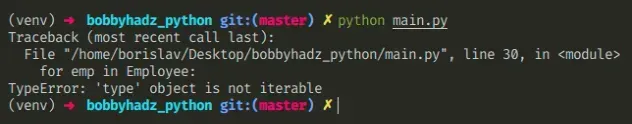Here is an example of how the error occurs when the `range` function.

main.py
```Copied!```# ⛔️ TypeError: 'type' object is not iterable
for i in range:
print(i)
``````

We forgot to call the `range` function which caused the error.

If you use the `range` function, make sure to call it.

main.py
```Copied!```for i in range(0, 3):  # 👉️ 0, 1, 2
print(i)
``````

The range class is commonly used for looping a specific number of times in `for` loops and takes the following parameters:

NameDescription
`start`An integer representing the start of the range (defaults to `0`)
`stop`Go up to, but not including the provided integer
`step`Range will consist of every N numbers from `start` to `stop` (defaults to `1`)

If you only pass a single argument to the `range()` constructor, it is considered to be the value for the `stop` parameter.

If values for the `start` and `stop` parameters are provided, the `start` value is inclusive, whereas the `stop` value is exclusive.

## Iterating over a class that doesn't implement the `__iter__` method#

You will also get the error if you try to iterate over a class that doesn't implement the `__iter__()` method.

main.py
```Copied!```class Counter:
pass

# ⛔️ TypeError: 'type' object is not iterable
for c in Counter:
print(c)
``````

If you need to make the class iterable, implement the `__iter__` method.

main.py
```Copied!```class Counter:
def __init__(self, start, stop):
self.current = start - 1
self.stop = stop

def __iter__(self):
return self

def __next__(self):
self.current += 1
if self.current < self.stop:
return self.current
raise StopIteration

for c in Counter(0, 4):
print(c)  # 👉️ 0, 1, 2, 3
``````

The `__iter__()` method is implicitly called at the start of loops and returns the iterator object.

The `__next__()` method is implicitly called at each loop increment and returns the next value.

## Checking if the object is iterable#

If you need to check if an object is iterable, use a `try/except` statement.

main.py
```Copied!```my_str = 'hello'

try:
my_iterator = iter(my_str)

for i in my_iterator:
print(i) # 👉️ h, e, l, l, o
except TypeError as te:
print(te)
``````

The iter() function raises a `TypeError` if the passed-in value doesn't support the `__iter__()` method or the sequence protocol (the `__getitem__()` method).

If we pass a non-iterable object like a class to the `iter()` function, the `except` block is run.

Examples of iterables include all sequence types (`list`, `str`, `tuple`) and some non-sequence types like `dict`, file objects and other objects that define an `__iter__()` or a `__getitem__()` method.

The Python "TypeError: 'type' object is not iterable" occurs when we try to iterate over a class that is not iterable, e.g. forget to call the `range()` function.

To solve the error, make the class iterable by implementing the `__iter__()` method.

I wrote a book in which I share everything I know about how to become a better, more efficient programmer.You can use the search field on my Home Page to filter through all of my articles.